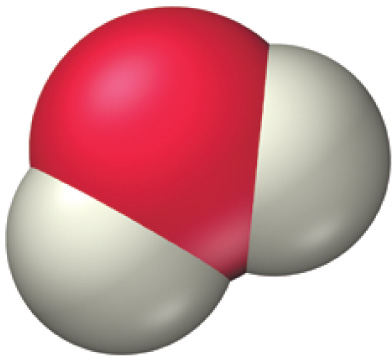# Problem: Throughout this textbook, we use space-filling molecular models to represent molecules. Which number is the best estimate for the scaling factor used in these models? In other words, by approximately what number would you have to multiply the radius of an actual oxygen atom to get the radius of the sphere used to represent the oxygen atom in the water molecule shown.

🤓 Based on our data, we think this question is relevant for Professor Mohammadi's class at CSULB.

###### FREE Expert Solution

atomic radii have hundreds of picometers

spheres in space-filling molecular structures have radii centimeter###### Problem DetailsThroughout this textbook, we use space-filling molecular models to represent molecules. Which number is the best estimate for the scaling factor used in these models? In other words, by approximately what number would you have to multiply the radius of an actual oxygen atom to get the radius of the sphere used to represent the oxygen atom in the water molecule shown.